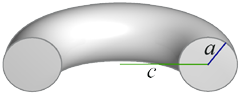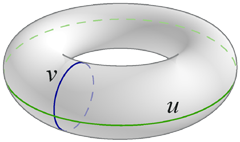]> The Torus

# The Torus

Not everything in life is simple

Following college courses in differential geometry and general relativity, I became interested in calculating different quantities associated with the curvature of surfaces. The standard examples are the sphere and cylinder, but they're not very interesting; their curvature is constant. I undertook an exploration of a slightly more complex surface, the torus.

## A Model of the Torus

We begin with a torus with major radius c and minor radius a. We assume a nice torus, such that c > a > 0 (a ring torus).We parameterize the torus's surface x in terms of coördinates u and v, each of which runs from 0 to 2π:$x u v = x = c + a cos v cos u y = c + a cos v sin u z = a sin v$

The origin of the coördinate system lies on the outer equator, where v = 0.

## Results

I used both tensor calculus and differential geometry to calculate a variety of quantities related to curvature. The full details are available in a 19-page PDF, but these pages summarize the most important results:

• Curvature: the torus's line element, metric, Christoffel symbols of the second kind, Riemann and Ricci tensors, and Ricci scalar.

• Geodesics: the five families of geodesics on the torus, and some open questions.

• Shape Operator: how the normal to the surface changes as we move on the surface.

• Parallel Transport: how vectors rotate as they are parallel transported on the surface.

Note that these pages omit intermediate steps. The PDF contains full derivations.

All this, and I don't particularly like doughnuts.

Last updated 15 November 2005
http://www.rdrop.com/~half/math/torus/index.xhtml
All contents ©2004 Mark L. Irons